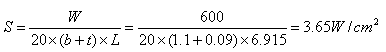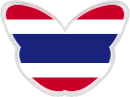## ติดต่อ Alloy Wire

120/55 ซอยสุขุมวิท 101/1 บางจาก พระโขนง จ.กรุงเทพฯ 10260

โทร: +66 (0) 80 626 9989
แฟกซ์: +66 (2) 398 6391
อีเมล: chompoonuut_t@alloywire.com# Designing a Tape ส่วนประกอบ

The method is similar to that used in designing a round wire element.

Where:

 b Tape width (mm) t Tape thickness (mm)

Example:

1.  To calculate the tape size and length required for a 600w/220v mica-wound element in a band heater, operating at a maximum temperature of 800°C, the total resistance of the element at operating temperature (Rt) will be: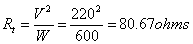2.  Using RW80 tape, the อุณหภูมิ Resistance Factor (F) at 800°C is 1.051.  Thus, the total resistance of the element at 20°C (R) will be: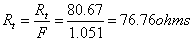3.  Knowing the dimensions of the mica-board, the length of the tape that may be wound round it may be estimated.  Thus, the resistance required per metre of tape will be:

For example a length of tape of 7 metres –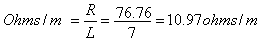4.  A tape size of 1.1mm x 0.09mm has a resistance per metre of 11.10 ohms/m (see stock sizes) which is near to 10.97 ohms/m.

5.  To verify the actual tape length (L)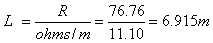A change in tape length may mean adding or subtracting turns (altering the pitch of the tape) to achieve the total resistance value required.